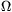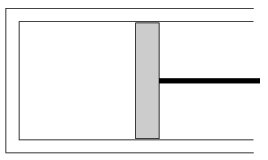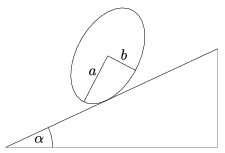Mathematical and Physical Journal
for High Schools
Issued by the MATFUND Foundation
 Already signed up? New to KöMaL?

# KöMaL Problems in Physics, January 2009

Show/hide problems of signs:## Problems with sign 'M'

Deadline expired on February 11, 2009.

M. 293. Measure how the resonant frequency of a plastic ruler (or some similar object), which is fixed at one of its ends, depends on the length of the ruler.

(6 pont)

statistics## Problems with sign 'P'

Deadline expired on February 11, 2009.

P. 4124. It is well known that a bowl of meat-soup cools down more quickly if there is a spoon in it. Why?

(3 pont)

statistics

P. 4125. It is often seen in crossroads where there is a traffic light that when the light shows green the front of a starting car rises up a bit. And also when the light goes red the back of a stopping car rises a bit. What is the explanation for these phenomena?

(3 pont)

statistics

P. 4126. A cube of uniform density is slid from one end of the table to the other. What is the maximum of the coefficient of the kinetic friction if the performed work is less than the work done while the cube is slowly rolled along its edges from one end of the table to the other?

(4 pont)

statistics

P. 4127. A battery of electromotive force 6 V and of internal resistance 8and a rechargeable battery of e.m.f. 6 V and of negligible internal resistance are connected in series.

a) What is the resistance of the resistor which is to be connected to the system in order to measure a voltage of 6 V across it?

b) What is the terminal voltage across the battery in this case?

(4 pont)

statistics

P. 4128. The pressure of the water supply of a city is maintained by a 24-m high water column. What is the daily electrical energy consumption of a city which has 50 000 citizens if the average water consumption of a citizen is 100 litres per day, the efficiency of the pumps is 80%, and the efficiency of the electric motors is 92%?

What is the average electrical power input of the water plant?

(4 pont)

statistics

P. 4129. The bob of a simple pendulum is displaced and then released. What can be the greatest angle of displacement, if the maximum force exerted by the thread is twice the weight of the bob, and the thread is not to be broken?

(4 pont)

statistics

P. 4130. The horizontal cylinder shown in the figure and the piston in it, which can move without friction, are adiabatically insulated. The mass of the piston is 10 kg, the area of its cross-section is 50 cm2. The temperature of the air confined in the cylinder is 27 oC, its initial pressure is equal to the atmospheric pressure. The cylinder is slowly turned into the vertical position. By what value does its temperature change?(5 pont)

statistics

P. 4131. Water which is at rest can be supercooled even to a temperature of -10 oC, without the water becoming a solid. If it is disturbed some part of it suddenly freezes. What is the amount of this suddenly freezing part?

(4 pont)

statistics

P. 4132. What is the amplitude of the electric field of the light emitted by a He-Ne laser whose power is 10 mW? The cross section of the beam is 1 cm2.

(5 pont)

statistics

P. 4133. What is the greatest angle of elevation of a slope onto which a cylinder which has an elliptical cross section can be placed, such that it stays at rest, provided that friction is big enough? The semi-major axis of the ellipse is a and its semi-minor axis is b.(5 pont)

statistics